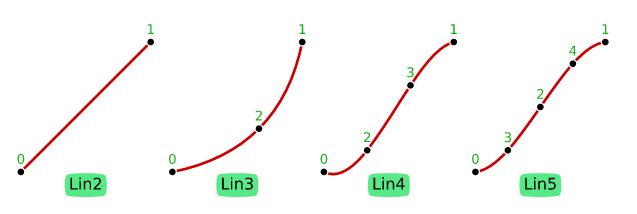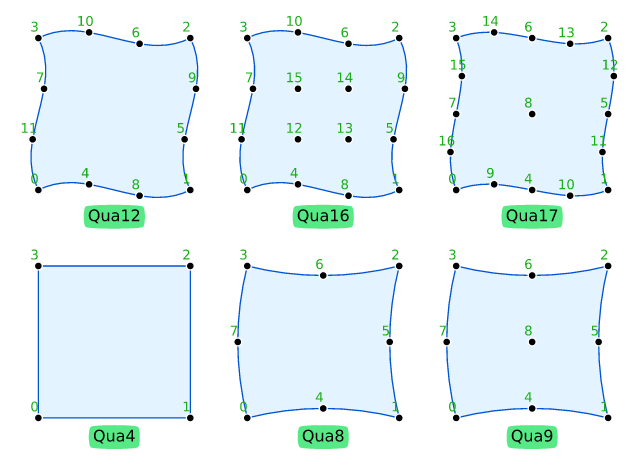## gemlab

Geometry and meshes laboratory for finite element analyses

### 5 releases(3 breaking)

 0.4.0 Jul 20, 2022 Jul 7, 2022 Jul 4, 2022 May 14, 2022 Oct 23, 2021

#325 in Math

Used in pmsim

4MB
25K SLoC

# Geometry, meshes, and numerical integration for finite element analyses

This repository contains structures and functions to perform geometry computations, generate meshes, and perform numerical integration for finite element analyses (FEM/FEA).

We use `Vector` and `Matrix` from Russell Lab, thus some Debian packages are required.

Documentation:

## Installation

Install some libraries:

``````sudo apt-get install \
liblapacke-dev \
libopenblas-dev
``````👆 Check the crate version and update your Cargo.toml accordingly:

``````[dependencies]
gemlab = "*"
``````

## Examples

``````use gemlab::integ::default_integ_points;
use gemlab::mesh::{set_pad_coords, At, Extract, Mesh, Region};
use gemlab::StrError;
use std::collections::HashSet;

fn main() -> Result<(), StrError> {
// Input the raw mesh data using a text file
// and compute all derived information such as shapes,
// and boundary entities. These data area stored in a
// Region for the sake of convenience.
//
// 1.0  5------,6.------7
//      | ,'   `. |
//      |  ,'       `.  |
//      |,'           `.|
// 0.5  3            4
//      |`.           .'|
//      |  `.       .'  |
//      | `.   .' |
// 0.0  0------`1'------2
//     0.0     0.5     1.0
let path = "./data/meshes/four_tri3_one_qua4.msh";
let mesh = Mesh::from_text_file(path)?;
let region = Region::with(&mesh, Extract::Boundary)?;

// Find entities along the boundary of the mesh
// by giving coordinates. The `At` enum provides
// an easy way to define the type of the constraint
// such as line, plane, circle, etc.
check(&region.find.points(At::Y(0.5))?, &[3, 4]);
check(&region.find.edges(At::X(1.0))?, &[(2, 4), (4, 7)]);

// Perform numerical integration to compute
// the area of cell # 2
let ndim = 2;
let cell_2 = &mesh.cells;
let ips = default_integ_points(cell_2.kind);
let mut area = 0.0;
for p in 0..ips.len() {
let iota = &ips[p];
let weight = ips[p];
area += weight * det_jac;
}
assert_eq!(area, 0.5);
Ok(())
}

fn check<T>(found: &HashSet<T>, correct: &[T])
where
T: Copy + Ord + std::fmt::Debug,
{
let mut ids: Vec<T> = found.iter().copied().collect();
ids.sort();
assert_eq!(ids, correct);
}
``````

## Todo

• Add tests for the numerical integrations
• Implement triangle and tetrahedron generators
• Implement drawing functions

## Available shapes and local numbering of nodes

### Lines -- Lin### Triangles -- Tri### Tetrahedra -- Tet### Hexahedra -- Hex### Geometry versus space dimensions

The following table shows what combinations of geometry-number-of-dimensions (`geo_ndim`) and space-number-of-dimensions (`space_ndim`) are possible. There are three cases:

1. Case `CABLE` -- `geo_ndim = 1` and `space_ndim = 2 or 3`; e.g., line in 2D or 3D (cables and rods)
2. Case `SHELL` -- `geo_ndim = 2` and `space_ndim = 3`; e.g. Tri or Qua in 3D (shells and surfaces)
3. Case `SOLID` -- `geo_ndim = space_ndim`; e.g., Tri and Qua in 2D or Tet and Hex in 3D
`geo_ndim` `space_ndim = 2` `space_ndim = 3`
1 `CABLE` `CABLE`
2 `SOLID` `SHELL`
3 impossible `SOLID`

### Coverage tool

The coverage tool cannot properly handle the macros in russell_chk such as assert_approx_eq! and assert_vec_approx_eq! We could use the `#[no_coverage]` decorator on the testing function to stop the coverage tool assessing the region coverage within the test function. However, we let the coverage tool report incorrect Region Coverage anyway. Sometimes, the coverage tool also fails to report line coverage even when all lines have been run.

~15MB
~287K SLoC